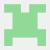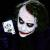java识别加减运算验证码

【福利】CTO直接拍板说好用的SDK全在这里了！0元配齐，全部免费！>>>0b

0public static int explanation(String exp) {
Pattern compile;
// 先乘除、再加减
if (exp.contains(Sign.DIVIDE.signChar) || exp.contains(Sign.MULTIPLY.signChar)) {
compile = Pattern.compile("(\\w+|\\d)(\\*|\\/)(\\w+|\\d)");
} else {
compile = Pattern.compile("(\\w+|\\d)(\\+|\\-)(\\w+|\\d)");
}
Matcher matcher = compile.matcher(exp);
if (matcher.find()) {
int val = Sign.get(matcher.group(2)).optional(Integer.valueOf(matcher.group(1)), Integer.valueOf(matcher.group(3)));
return explanation(matcher.replaceFirst(String.valueOf(val)));
}
return Integer.valueOf(exp);
}

enum Sign {

PLUS("+") {
@Override
public int optional(int a, int b) {
return a+b;
}
},

SUB("-"){
@Override
public int optional(int a, int b) {
return a-b;
}
},

MULTIPLY("*"){
@Override
public int optional(int a, int b) {
return a*b;
}
},

DIVIDE("/"){
@Override
public int optional(int a, int b) {
return a/b;
}
};

private String signChar;

Sign(String signChar) {
this.signChar = signChar;
}

public static Sign get(String singChar) {
for (Sign sign : values()) {
if (singChar.equals(sign.signChar)){
return sign;
}
}
return null;
}

public abstract int optional(int a, int b);
}